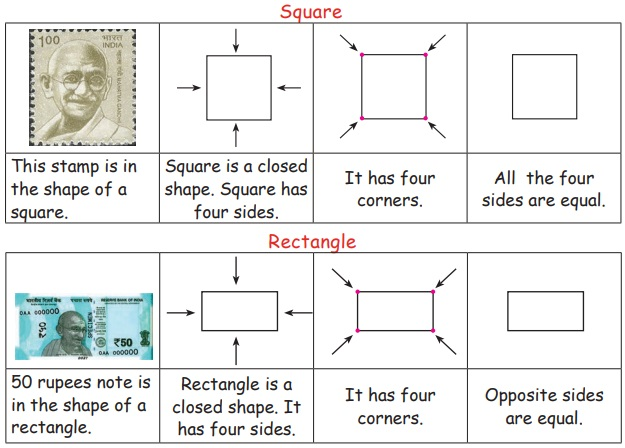Home | | Maths 2nd Std | Properties of 2D shapes

# Properties of 2D shapes

Teacher’s Note : Teacher narrates the story of sheep and fox to enhance the usage of the words such as close and open.

UNIT  1

GeometryProperties of 2D shapes

Travel Through

Ask the students to form a circle by holding their hands. Then call any three students to act like lamb, sheep and fox. Make them play the game as follows.Keywords: Open shape, Closed shape

Teacher’s Note : Teacher narrates the story of sheep and fox to enhance the usage of the words such as close and open.

Learn

Properties of 2D shapes

Let us learn the properties of 2D shapes.Square

This stamp is in the shape of a square.

Square is a closed shape. Square has four sides

It has four corners.

All the four sides are equal.

Rectangle

50 rupees note is in the shape of a rectangle.

Rectangle is a closed shape. It has four sides.

It has four corners.

Opposite sides are equal.

Triangle

Traffic signal is in the shape of a triangle.

Triangle is a closed shape. It has three sides.

It has three corners.

Sides can be equal or unequal.

Circle

Coin is in the shape of a circle.

Circle is a closed curve.

Practice

Write “C” for closed shapes and “O” for open shapes in the given space.Complete the table.Try This

Observe the shape in the geoboard and draw as many similar shapes of various sizes in the dot sheet given on the right side of it.Teacher’s Note: Teacher can facilitate children to form 2D shapes in geoboard using rubberbands.

Think Like a Mathematician

Can you form a circle using rubberband in a geoboard?

Yes, can.

Tags : Geometry | Term 2 Chapter 1 | 2nd Maths , 2nd Maths : Term 2 Unit 1 : Geometry
Study Material, Lecturing Notes, Assignment, Reference, Wiki description explanation, brief detail
2nd Maths : Term 2 Unit 1 : Geometry : Properties of 2D shapes | Geometry | Term 2 Chapter 1 | 2nd Maths